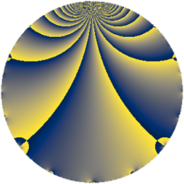# Properties

 Label 80.1.h.aLevel $80$ Weight $1$ Character orbit 80.h Self dual yes Analytic conductor $0.040$ Analytic rank $0$ Dimension $1$ Projective image $D_{2}$ CM/RM discs -4, -20, 5 Inner twists $4$

# Related objects

Show commands: Magma / PariGP / SageMath

## Newspace parameters

comment: Compute space of new eigenforms

[N,k,chi] = [80,1,Mod(79,80)]

mf = mfinit([N,k,chi],0)

lf = mfeigenbasis(mf)

from sage.modular.dirichlet import DirichletCharacter

H = DirichletGroup(80, base_ring=CyclotomicField(2))

chi = DirichletCharacter(H, H._module([1, 0, 1]))

N = Newforms(chi, 1, names="a")

//Please install CHIMP (https://github.com/edgarcosta/CHIMP) if you want to run this code

chi := DirichletCharacter("80.79");

S:= CuspForms(chi, 1);

N := Newforms(S);

 Level: $$N$$ $$=$$ $$80 = 2^{4} \cdot 5$$ Weight: $$k$$ $$=$$ $$1$$ Character orbit: $$[\chi]$$ $$=$$ 80.h (of order $$2$$, degree $$1$$, minimal)

## Newform invariants

comment: select newform

sage: f = N # Warning: the index may be different

gp: f = lf \\ Warning: the index may be different

 Self dual: yes Analytic conductor: $$0.0399252010106$$ Analytic rank: $$0$$ Dimension: $$1$$ Coefficient field: $$\mathbb{Q}$$ Coefficient ring: $$\mathbb{Z}$$ Coefficient ring index: $$1$$ Twist minimal: yes Projective image: $$D_{2}$$ Projective field: Galois closure of $$\Q(i, \sqrt{5})$$ Artin image: $D_4$ Artin field: Galois closure of 4.0.320.1

## $q$-expansion

comment: q-expansion

sage: f.q_expansion() # note that sage often uses an isomorphic number field

gp: mfcoefs(f, 20)

 $$f(q)$$ $$=$$ $$q - q^{5} - q^{9}+O(q^{10})$$ q - q^5 - q^9 $$q - q^{5} - q^{9} + q^{25} + 2 q^{29} - 2 q^{41} + q^{45} - q^{49} - 2 q^{61} + q^{81} + 2 q^{89}+O(q^{100})$$ q - q^5 - q^9 + q^25 + 2 * q^29 - 2 * q^41 + q^45 - q^49 - 2 * q^61 + q^81 + 2 * q^89

## Expression as an eta quotient

$$f(z) = \eta(4z)\eta(20z)=q\prod_{n=1}^\infty(1 - q^{4n})^{}(1 - q^{20n})^{}$$

## Character values

We give the values of $$\chi$$ on generators for $$\left(\mathbb{Z}/80\mathbb{Z}\right)^\times$$.

 $$n$$ $$17$$ $$21$$ $$31$$ $$\chi(n)$$ $$-1$$ $$1$$ $$-1$$

## Embeddings

For each embedding $$\iota_m$$ of the coefficient field, the values $$\iota_m(a_n)$$ are shown below.

For more information on an embedded modular form you can click on its label.

comment: embeddings in the coefficient field

gp: mfembed(f)

Label $$\iota_m(\nu)$$ $$a_{2}$$ $$a_{3}$$ $$a_{4}$$ $$a_{5}$$ $$a_{6}$$ $$a_{7}$$ $$a_{8}$$ $$a_{9}$$ $$a_{10}$$
79.1
 0
0 0 0 −1.00000 0 0 0 −1.00000 0
 $$n$$: e.g. 2-40 or 990-1000 Significant digits: Format: Complex embeddings Normalized embeddings Satake parameters Satake angles

## Inner twists

Char Parity Ord Mult Type
1.a even 1 1 trivial
4.b odd 2 1 CM by $$\Q(\sqrt{-1})$$
5.b even 2 1 RM by $$\Q(\sqrt{5})$$
20.d odd 2 1 CM by $$\Q(\sqrt{-5})$$

## Twists

By twisting character orbit
Char Parity Ord Mult Type Twist Min Dim
1.a even 1 1 trivial 80.1.h.a 1
3.b odd 2 1 720.1.j.a 1
4.b odd 2 1 CM 80.1.h.a 1
5.b even 2 1 RM 80.1.h.a 1
5.c odd 4 2 400.1.b.a 1
7.b odd 2 1 3920.1.j.a 1
7.c even 3 2 3920.1.bt.b 2
7.d odd 6 2 3920.1.bt.a 2
8.b even 2 1 320.1.h.a 1
8.d odd 2 1 320.1.h.a 1
12.b even 2 1 720.1.j.a 1
15.d odd 2 1 720.1.j.a 1
15.e even 4 2 3600.1.e.a 1
16.e even 4 2 1280.1.e.a 2
16.f odd 4 2 1280.1.e.a 2
20.d odd 2 1 CM 80.1.h.a 1
20.e even 4 2 400.1.b.a 1
24.f even 2 1 2880.1.j.a 1
24.h odd 2 1 2880.1.j.a 1
28.d even 2 1 3920.1.j.a 1
28.f even 6 2 3920.1.bt.a 2
28.g odd 6 2 3920.1.bt.b 2
35.c odd 2 1 3920.1.j.a 1
35.i odd 6 2 3920.1.bt.a 2
35.j even 6 2 3920.1.bt.b 2
40.e odd 2 1 320.1.h.a 1
40.f even 2 1 320.1.h.a 1
40.i odd 4 2 1600.1.b.a 1
40.k even 4 2 1600.1.b.a 1
60.h even 2 1 720.1.j.a 1
60.l odd 4 2 3600.1.e.a 1
80.k odd 4 2 1280.1.e.a 2
80.q even 4 2 1280.1.e.a 2
120.i odd 2 1 2880.1.j.a 1
120.m even 2 1 2880.1.j.a 1
140.c even 2 1 3920.1.j.a 1
140.p odd 6 2 3920.1.bt.b 2
140.s even 6 2 3920.1.bt.a 2

By twisted newform orbit
Twist Min Dim Char Parity Ord Mult Type
80.1.h.a 1 1.a even 1 1 trivial
80.1.h.a 1 4.b odd 2 1 CM
80.1.h.a 1 5.b even 2 1 RM
80.1.h.a 1 20.d odd 2 1 CM
320.1.h.a 1 8.b even 2 1
320.1.h.a 1 8.d odd 2 1
320.1.h.a 1 40.e odd 2 1
320.1.h.a 1 40.f even 2 1
400.1.b.a 1 5.c odd 4 2
400.1.b.a 1 20.e even 4 2
720.1.j.a 1 3.b odd 2 1
720.1.j.a 1 12.b even 2 1
720.1.j.a 1 15.d odd 2 1
720.1.j.a 1 60.h even 2 1
1280.1.e.a 2 16.e even 4 2
1280.1.e.a 2 16.f odd 4 2
1280.1.e.a 2 80.k odd 4 2
1280.1.e.a 2 80.q even 4 2
1600.1.b.a 1 40.i odd 4 2
1600.1.b.a 1 40.k even 4 2
2880.1.j.a 1 24.f even 2 1
2880.1.j.a 1 24.h odd 2 1
2880.1.j.a 1 120.i odd 2 1
2880.1.j.a 1 120.m even 2 1
3600.1.e.a 1 15.e even 4 2
3600.1.e.a 1 60.l odd 4 2
3920.1.j.a 1 7.b odd 2 1
3920.1.j.a 1 28.d even 2 1
3920.1.j.a 1 35.c odd 2 1
3920.1.j.a 1 140.c even 2 1
3920.1.bt.a 2 7.d odd 6 2
3920.1.bt.a 2 28.f even 6 2
3920.1.bt.a 2 35.i odd 6 2
3920.1.bt.a 2 140.s even 6 2
3920.1.bt.b 2 7.c even 3 2
3920.1.bt.b 2 28.g odd 6 2
3920.1.bt.b 2 35.j even 6 2
3920.1.bt.b 2 140.p odd 6 2

## Hecke kernels

This newform subspace is the entire newspace $$S_{1}^{\mathrm{new}}(80, [\chi])$$.

## Hecke characteristic polynomials

$p$ $F_p(T)$
$2$ $$T$$
$3$ $$T$$
$5$ $$T + 1$$
$7$ $$T$$
$11$ $$T$$
$13$ $$T$$
$17$ $$T$$
$19$ $$T$$
$23$ $$T$$
$29$ $$T - 2$$
$31$ $$T$$
$37$ $$T$$
$41$ $$T + 2$$
$43$ $$T$$
$47$ $$T$$
$53$ $$T$$
$59$ $$T$$
$61$ $$T + 2$$
$67$ $$T$$
$71$ $$T$$
$73$ $$T$$
$79$ $$T$$
$83$ $$T$$
$89$ $$T - 2$$
$97$ $$T$$